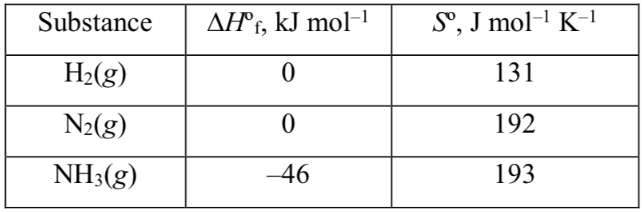# Problem: What is the standard Gibbs free energy of formation, ΔG°f, of NH3(g) at 298 K?

###### FREE Expert Solution

Reaction: N2(g) + 3 H2(g) → 2 NH3(g)

Step 1: We can use the following equation to solve for ΔH˚rxn:

Note that we need to multiply each ΔH˚f by the stoichiometric coefficient since ΔH˚f is in kJ/mol.

Also, note that ΔH˚f for elements in their standard state is 0.

We also need to convert ΔH˚rxn from kJ/mol to J/ mol so our units remain consistent.

ΔH˚rxn = –92000 J

Step 2: We can use the following equation to solve for ΔS˚rxn:

Note that we need to multiply each S˚ by the stoichiometric coefficient since S˚ is in J/mol • K.

ΔS˚rxn = –199 J/K###### Problem Details

What is the standard Gibbs free energy of formation, ΔG°f, of NH3(g) at 298 K?